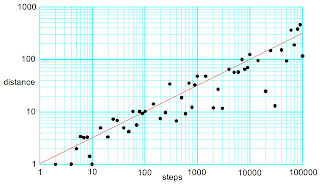## Friday, July 13, 2018

### Diffusion as a Random Walk

Intermediate Physics for Medicine and Biology: Diffusion as a Random Walk At the end of Chapter 4 of Intermediate Physics for Medicine and Biology, Russ Hobbie and I model diffusion as a random walk.
The spreading solution to the one-dimensional diffusion equation that we verified can also be obtained by treating the motion of a molecule as a series of independent steps either to the right or to the left along the x axis.
Figure 4.24 in IPMB shows a simulation of a two-dimensional random walk that Russ added to the second edition.
Note how the particle wanders around one region of space and then takes a number of steps in the same direction to move someplace else. The particle trajectory is “thready.” It does not cover space uniformly. A uniform coverage would be very nonrandom. It is only when many particles are considered that a Gaussian distribution of particle concentration results.
I thought readers would profit from seeing the results of several simulations, so they won’t draw too many conclusions from one sample. Also, why let Russ have all the fun? So I wrote this MATLAB code, where “rand” is a random number generator with output between zero and one.MATLAB code to perform a two-dimensional random walk.
Below I show nine different particle trajectories (plots of y versus x), for 40,000 steps (the same number Russ used in IPMB). The red dot indicates the starting location and the blue path shows the particle trajectory, which does look “thready.”The particle trajectory for nine samples of a two-dimensional random walk, each with 40,000 steps.
I also performed simulations for different numbers of steps, where r is the mean distance from the starting point to the end of the trajectory calculated by averaging over 10,000 samples. The red line is the result from continuum theory: distance equals the square root of the number of steps. The calculations agree with the theoretical prediction, but there is much scatter.The average distance of the particle from the starting point as a function of the number of steps, for a two-dimensional random walk.
In IPMB we include an analogous result form Russ’s calculation, in which he averaged over only 328 samples, each with 10,000 steps. His results were within about a tenth of a percent of the theoretical prediction. Given the scatter in my simulations, I’m guessing Russ got lucky.